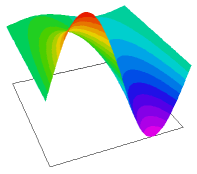﻿ Sample Problems > Usage > Misc > spacetime1

spacetime1

Navigation:  Sample Problems > Usage > Misc >

spacetime1{ SPACETIME1.PDE

This example illustrates the use of FlexPDE to solve an initial value problem

of 1-D transient heatflow as a 2D boundary-value problem.

Here the spatial coordinate is represented by X, the time coordinate by Y,

and the temperature by u(x,y).

With these symbols, the transient heatflow equation is:

dy(u) = D*dxx(u),

where D is the diffusivity, given by

D = K/s*rho,

K       is the conductivity,

s       is the specific heat,

and   rho     is the density.

The problem domain is taken to be the unit square.

We specify the initial value of u(x,0) along y=0, as well as the time history

along the sides x=0 and x=1.

The value of u is thus assigned everywhere on the boundary except

along the segment y=1, 0<x<1.  Along that boundary, we use the

natural boundary condition,

natural(u) = 0,

since this corresponds to the application of no boundary sources on this

boundary segment and hence implies a free segment.  This builds in the

assumption that y = 1 (and hence t = 1) is sufficiently large for steady

state to have been reached. [Note that since the only y-derivative term is

first order, the default procedure of FlexPDE does not integrate this term

by parts, and the Natural(u) BC does not correspond to a surface flux,

functioning only as a source or sink.]

This problem can be solved analytically, so we can plot the deviation

of the FlexPDE solution from the exact answer.

}

title "1-D Transient Heatflow as a Boundary-Value problem"

select

alias(x) "distance"

alias(y) "time"

variables

u

definitions

diffusivity = 0.06    { pick a diffusivity that gives a nice graph }

frequency = 2         { frequency of initial sinusoid }

fpi = frequency*pi

ut0 = sin(fpi*x)     { define initial distribution of temperature }

u0 = exp(-fpi^2 *diffusivity*y)*ut0   { define exact solution }

Initial values

u = ut0              { initialize all time to t=0 value }

equations

U: dy(u) = diffusivity*dxx(u) { define the heatflow equation }

 boundaries     Region 1       start(0,0)       value(u)=ut0    { set the t=0 temperature }       line to (1,0)       value(u) = 0   { always cold at x=1 }       line to (1,1)       natural(u) = 0 { no sources at t=1 }       line to (0,1)       value(u) = 0   { always cold at x=0 }       line to close monitors     contour(u) plots     contour(u)     surface(u)     contour(u-u0) as "error" end